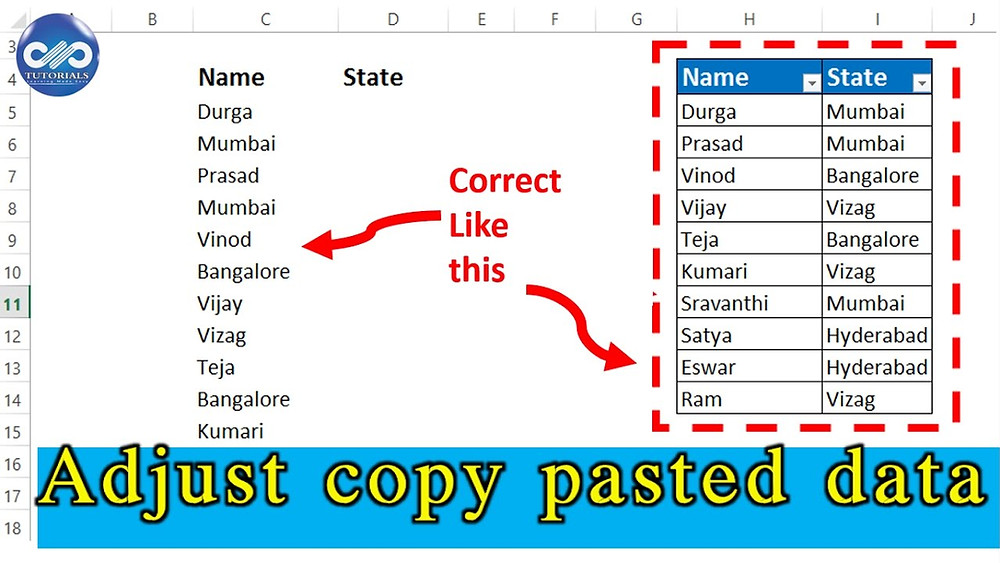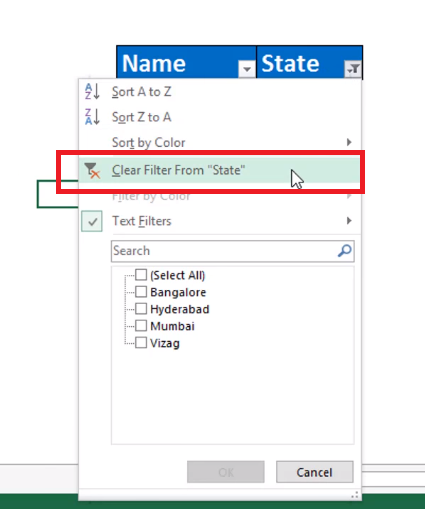Search

# Adjust Copy Pasted Excel Data into Correct ColumnsIn this tutorial, you will learn how to clean the excel data into the right matrix.

Sometimes when you copy paste the data from other sources, all the pasted data comes in a disordered manner, into a single column .Now let us see how to arrange the data into correct columns using the formula.

In this example, let us consider data of some people and their living places, which has come into a single column after extracting from some source.

Now let us arrange this data by name and their corresponding State name adjacent to each other.

Before we get into the exact process of cleaning up, Just make sure that our first entry of the data range starts from the odd row number, here it is 5th Row.

Here, I would be using combination of two function ROW and MOD.

As discussed earlier, ROW function gives the row number of the cell selected and MOD gives the remainder of the number.

Step 1: Copy the entire Data from Column C and paste it from cell H5.

Step 2: In cell I5, type the formula as

=IF(MOD(ROW(H5),2)=1,H6,"")here, ROW(H5) gives the row number of cell H5, i.e. 5

MOD(5,2) gives the remainder as 1 and by IF condition, as it is true it should give the result as H6, if false it will show the result as blank.

Now press enter and see the result as "MUMBAI" which is cell H6.Step 3: Drag this formula from cell H6 to bottom of the table, to see the working of the formula.Step 4: Now, you need to just delete all the rows containing blank cells in column I.

Copy and paste the fields as values and apply filter in column I to filter blank cells and then delete rows.Step 5: Clear filters now.So, In this way, we can correct and clean the data in a smarter way.Watch this video tutorial for better understanding:

If you liked this tutorial, share it with your friends. And also you can follow us on Youtube, Twitter and Facebook. We would love to hear from you, Please do comment, suggest or compliment our work and we shall make it better for you. You can write us at dptutorials15@gmail.com